# DDA Algorithm | Line Drawing Algorithms

## Line Drawing Algorithms-

In computer graphics, popular algorithms used to generate lines are-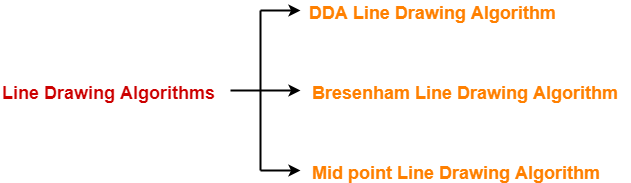1. Digital Differential Analyzer (DDA) Line Drawing Algorithm
2. Bresenham Line Drawing Algorithm
3. Mid Point Line Drawing Algorithm

## DDA Algorithm-

DDA Algorithm is the simplest line drawing algorithm.

 Given the starting and ending coordinates of a line, DDA Algorithm attempts to generate the points between the starting and ending coordinates.

### Procedure-

Given-

• Starting coordinates = (X0, Y0)
• Ending coordinates = (Xn, Yn)

The points generation using DDA Algorithm involves the following steps-

### Step-01:

Calculate ΔX, ΔY and M from the given input.

These parameters are calculated as-

• ΔX = Xn – X0
• ΔY =Yn – Y0
• M = ΔY / ΔX

### Step-02:

Find the number of steps or points in between the starting and ending coordinates.

if (absolute (ΔX) > absolute (ΔY))

Steps = absolute (ΔX);

else

Steps = absolute (ΔY);

### Step-03:

Suppose the current point is (Xp, Yp) and the next point is (Xp+1, Yp+1).

Find the next point by following the below three cases-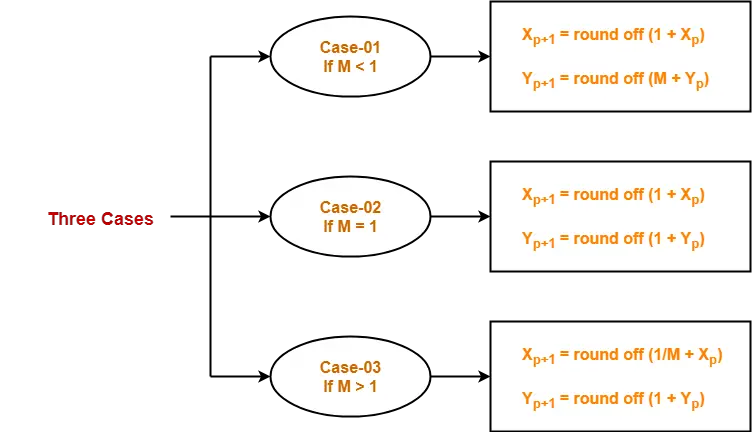### Step-04:

Keep repeating Step-03 until the end point is reached or the number of generated new points (including the starting and ending points) equals to the steps count.

## Problem-01:

Calculate the points between the starting point (5, 6) and ending point (8, 12).

## Solution-

Given-

• Starting coordinates = (X0, Y0) = (5, 6)
• Ending coordinates = (Xn, Yn) = (8, 12)

### Step-01:

Calculate ΔX, ΔY and M from the given input.

• ΔX = Xn – X0 = 8 – 5 = 3
• ΔY =Yn – Y0 = 12 – 6 = 6
• M = ΔY / ΔX = 6 / 3 = 2

### Step-02:

Calculate the number of steps.

As |ΔX| < |ΔY| = 3 < 6, so number of steps = ΔY = 6

### Step-03:

As M > 1, so case-03 is satisfied.

Now, Step-03 is executed until Step-04 is satisfied.

 Xp Yp Xp+1 Yp+1 Round off (Xp+1, Yp+1) 5 6 5.5 7 (6, 7) 6 8 (6, 8) 6.5 9 (7, 9) 7 10 (7, 10) 7.5 11 (8, 11) 8 12 (8, 12)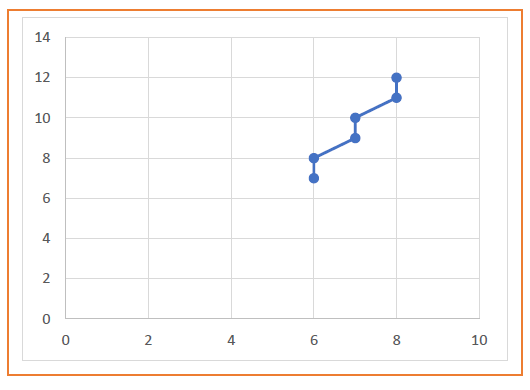## Problem-02:

Calculate the points between the starting point (5, 6) and ending point (13, 10).

## Solution-

Given-

• Starting coordinates = (X0, Y0) = (5, 6)
• Ending coordinates = (Xn, Yn) = (13, 10)

### Step-01:

Calculate ΔX, ΔY and M from the given input.

• ΔX = Xn – X0 = 13 – 5 = 8
• ΔY =Yn – Y0 = 10 – 6 = 4
• M = ΔY / ΔX = 4 / 8 = 0.50

### Step-02:

Calculate the number of steps.

As |ΔX| > |ΔY| = 8 > 4, so number of steps = ΔX = 8

### Step-03:

As M < 1, so case-01 is satisfied.

Now, Step-03 is executed until Step-04 is satisfied.

 Xp Yp Xp+1 Yp+1 Round off (Xp+1, Yp+1) 5 6 6 6.5 (6, 7) 7 7 (7, 7) 8 7.5 (8, 8) 9 8 (9, 8) 10 8.5 (10, 9) 11 9 (11, 9) 12 9.5 (12, 10) 13 10 (13, 10)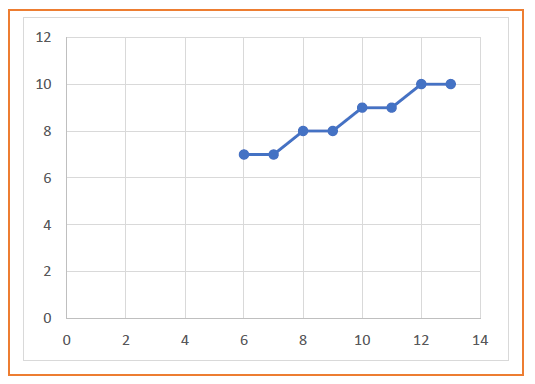## Problem-03:

Calculate the points between the starting point (1, 7) and ending point (11, 17).

## Solution-

Given-

• Starting coordinates = (X0, Y0) = (1, 7)
• Ending coordinates = (Xn, Yn) = (11, 17)

### Step-01:

Calculate ΔX, ΔY and M from the given input.

• ΔX = Xn – X0 = 11 – 1 = 10
• ΔY =Yn – Y0 = 17 – 7 = 10
• M = ΔY / ΔX = 10 / 10 = 1

### Step-02:

Calculate the number of steps.

As |ΔX| = |ΔY| = 10 = 10, so number of steps = ΔX = ΔY = 10

### Step-03:

As M = 1, so case-02 is satisfied.

Now, Step-03 is executed until Step-04 is satisfied.

 Xp Yp Xp+1 Yp+1 Round off (Xp+1, Yp+1) 1 7 2 8 (2, 8) 3 9 (3, 9) 4 10 (4, 10) 5 11 (5, 11) 6 12 (6, 12) 7 13 (7, 13) 8 14 (8, 14) 9 15 (9, 15) 10 16 (10, 16) 11 17 (11, 17)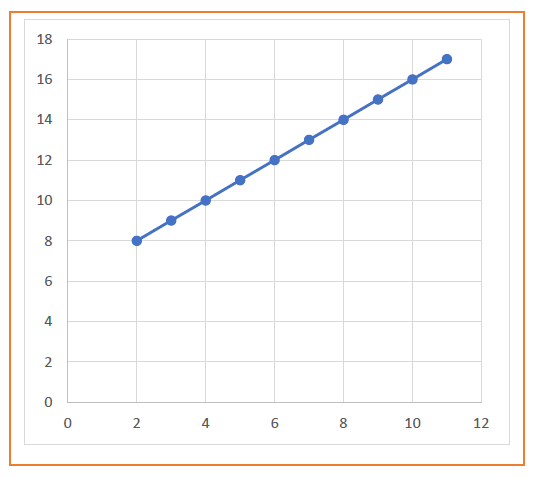The advantages of DDA Algorithm are-

• It is a simple algorithm.
• It is easy to implement.
• It avoids using the multiplication operation which is costly in terms of time complexity.

The disadvantages of DDA Algorithm are-

• There is an extra overhead of using round off( ) function.
• Using round off( ) function increases time complexity of the algorithm.
• Resulted lines are not smooth because of round off( ) function.
• The points generated by this algorithm are not accurate.

To gain better understanding about DDA Algorithm,

Watch this Video Lecture

Next Article- Bresenham Line Drawing Algorithm

Get more notes and other study material of Computer Graphics.

Watch video lectures by visiting our YouTube channel LearnVidFun.

SummaryArticle Name
DDA Algorithm | Line Drawing Algorithms
Description
Line Drawing Algorithms- In computer graphics, DDA Line Drawing Algorithm is the simplest line drawing algorithm. DDA Line Drawing Algorithm attempts to generate the points between the starting and ending coordinates.
Author
Publisher Name
Gate Vidyalay
Publisher Logo